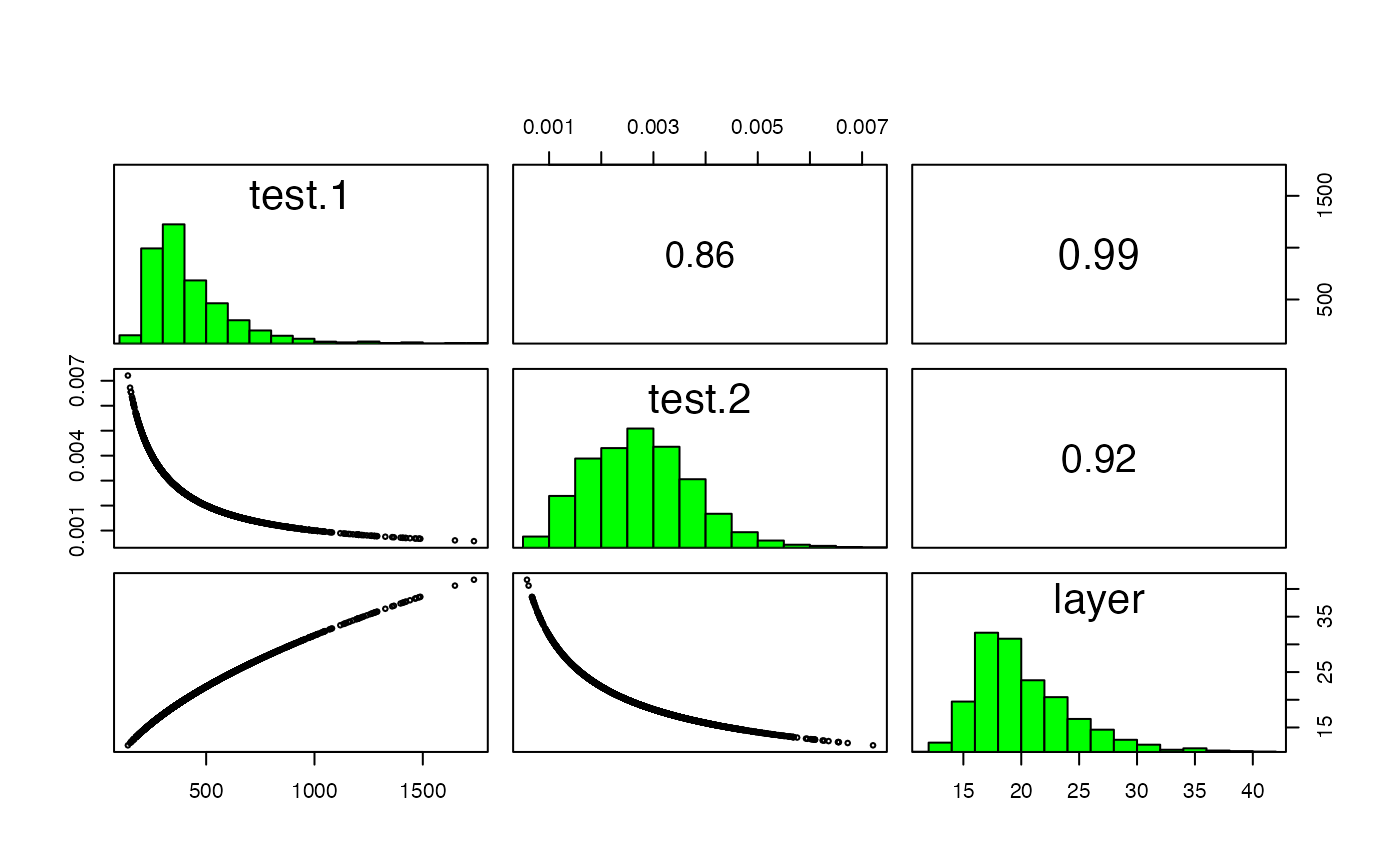Pair plots of layers in a RasterStack or RasterBrick. This is a wrapper around graphics function pairs.

# S4 method for RasterStackBrick
pairs(x, hist=TRUE, cor=TRUE, use="pairwise.complete.obs", maxpixels=100000, ...)

## Arguments

x

RasterBrick or RasterStack

hist

Logical. If TRUE a histogram of the values is shown on the diagonal

cor

Logical. If TRUE the correlation coefficient is shown in the upper panels

use

Argument passed to the cor function

maxpixels

Integer. Number of pixels to sample from each layer of large Raster objects

...

Additional arguments (only cex and main)

boxplot, hist, density

## Examples

r <- raster(system.file("external/test.grd", package="raster") )
s <- stack(r, 1/r, sqrt(r))
pairs(s)if (FALSE) {
# to make indvidual histograms:
hist(r)
# or scatter plots:
plot(r, 1/r)
}